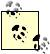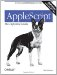Section 9.9. Recursion

9.9. Recursion

A handler is visible from within itself. This means that recursion is possible; a handler may call itself.

Explaining the elegances and dangers of recursion is beyond the scope of this book. The best way to learn about recursion is through a language like Scheme or LISP , where recursion is the primary form of looping. In fact, in conjunction with lists, AppleScript's recursion allows some remarkably Scheme-like (or LISP-like) modes of expression (see "LISP-likeness " in Chapter 4).The best way to learn Scheme is to read Harold Abelson et al., Structure and Interpretation of Computer Programs, 2nd Edition (MIT Press, 1996), the best computer book ever written.

For example, here's a recursive routine for filtering a list. We'll remove from the list everything that isn't a number:

on filter(L)     if L = {} then return L     if {class of item 1 of L} is in {real, integer, number} then         return {item 1 of L} & filter(rest of L)     else         return filter(rest of L)     end if end filter filter({"hey", 1, "ho", 2, 3}) -- {1, 2, 3}

AppleScript is not a truly recursive language, however; recursion is limited by the depth of AppleScript's internal stack. If you recurse too deep (which usually means recursing through too long a list), you'll get a "stack overflow " error message. Unfortunately there's no way to know in advance what the limit is, as it depends on what happens during each recursion and on what environment is running the script. Just to give a typical example, using Script Editor on my machine, this code runs fine if max is 504 but fails with a stack overflow if max is 505; but in Smile it works even if max is as large as 8159:

on remvix(L, ix)     if L is {} then return {}     if ix is 1 then         return rest of L     else         return item 1 of L & remvix(rest of L, ix - 1)     end if end remvix set L to {} set max to 505 repeat with x from 1 to max     set end of L to x end repeat remvix(L, max)

Be careful when assigning a recursive handler as a value to a variable. At the point where the handler calls itself, its name is hard-coded into its functionality. After assigning the handler functionality to a different variable name, that name may no longer be in scope and the handler will break when called under its new name:

script s     on filter(L)         if L = {} then return L         if {class of item 1 of L} is in {real, integer, number} then             return {item 1 of L} & filter(rest of L)         else             return filter(rest of L)         end if     end filter end script set f to s's filter f({"hey", 1, "ho", 2, 3}) -- error: «script» doesn't understand the filter messageAppleScript: The Definitive Guide, 2nd Edition
ISBN: 0596102119
EAN: 2147483647
Year: 2006
Pages: 267
Authors: Matt Neuburg

Similar book on Amazon